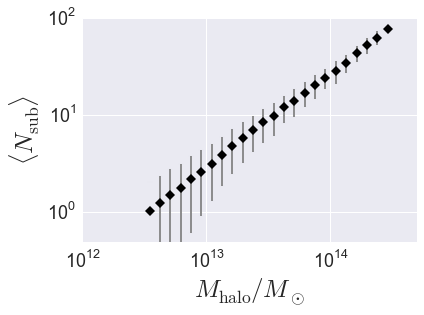# Halo Catalog Analysis Example: halo properties as a function of host halo mass¶

In this example, we’ll show how to start from a subhalo catalog and calculate how various properties scale with host halo mass. As a specific example, we’ll calculate the average abundance of subhalos as a function of mass, $$\langle N_{\rm sub}\vert M_{\rm halo}\rangle$$.

There is also an IPython Notebook in the following location that can be used as a companion to the material in this section of the tutorial:

halotools/docs/notebooks/halocat_analysis/basic_examples/halo_catalog_analysis_tutorial1.ipynb

By following this tutorial together with this notebook, you can play around with your own variations of the calculation as you learn the basic syntax.

## Retrieve the default halo catalog¶

from halotools.sim_manager import CachedHaloCatalog
halocat = CachedHaloCatalog()
print(halocat.halo_table[0:9])

halo_vmax_firstacc halo_dmvir_dt_tdyn ... halo_hostid halo_mvir_host_halo
------------------ ------------------ ... ----------- -------------------
67.3             -5.505 ...  3058439856           2.031e+10
99.91             -9.513 ...  3058439861           4.443e+10
87.86             0.8171 ...  3058439904           9.882e+10
78.43             -1.356 ...  3058439906           3.108e+10
89.69              1.495 ...  3058439907           4.266e+10
118.89             -6.333 ...  3058439910           1.728e+11
123.38              4.487 ...  3058439952           1.867e+11
109.28             -15.28 ...  3058439956           6.897e+10
84.17            -0.2037 ...  3058439985           3.339e+10


The first time you load the halo catalog into memory is slow because the halo table is sorted into a convenient order and a large number of self-consistency checks are performed. Subsequent calls to extract the halo_table are fast as the catalog is now available in RAM.

mask = halocat.halo_table['halo_mpeak'] > 1000*halocat.particle_mass


## Calculate total number of subhalos $$N_{\rm subs}$$ in each halo¶

To calculate the total number of subhalos in each host halo, we’ll use the halotools.utils.group_member_generator. You can read more about the details of the generator in its documentation, here we’ll just demo some basic usage. Briefly, this generator can be used to iterate over your halo population on a host-by-host basis, so that you can perform group-wise calculations with each step of the iteration. In this case, at each step of the iteration we’ll sum up the total number of subhalos associated with each host.

As described in Rockstar halo and subhalo nomenclature conventions, halo_hostid is a natural grouping key for a subhalo catalog. So we’ll sort our subhalo catalog on this column and notify the group_member_generator that we have done so by passing in halo_hostid as the grouping_key keyword argument. For this calculation, all we care about is the number of objects within each grouping, so there is no need to request the group_member_generator to yield any particular data about the subhalos, so we can simply set the requested_columns argument to be an empty list. After calling the generator with these arguments, we will then proceed to loop over the generator, calculate the number of subhalos in each host, and at the end store the result in a new column of the halo table.

from halotools.utils import group_member_generator

halos.sort('halo_hostid')
grouping_key = 'halo_hostid'
requested_columns = []
group_gen = group_member_generator(halos, grouping_key, requested_columns)

nsub = np.zeros(len(halos))
for first, last, member_props in group_gen:
nsub[first:last] = last - first - 1

halos['num_subhalos'] = nsub


Our halos table now has a num_subhalos column.

## Calculate $$\langle N_{\rm sub}\rangle$$ vs. $$M_{\rm halo}$$¶

Now we’ll exploit our previous calculations to compute the mean number of subhalos in bins of halo mass. For this calculation, the mean_y_vs_x provides useful wrapper behavior around scipy.stats.binned_statistic and numpy.histogram. Note that mean_y_vs_x is really just a convenience function used for quick exploratory work. For results going into science publications, be sure to check how your findings depend on bin width, sampling, etc.

from halotools.mock_observables import mean_y_vs_x
import numpy as np

bins = np.logspace(12.5, 14.5, 25)
result = mean_y_vs_x(hosts['halo_mvir_host_halo'], hosts['num_subhalos'],
bins = bins, error_estimator = 'variance')

host_mass, mean_richness, richness_variance = result


### Plot the result¶

from seaborn import plt

plt.errorbar(host_mass, mean_richness, yerr=richness_variance,
fmt = "none", ecolor='gray')
plt.plot(host_mass, mean_richness, 'D', color='k')

plt.loglog()
plt.xticks(size=18)
plt.yticks(size=18)
plt.xlabel(r'$M_{\rm halo}/M_{\odot}$', fontsize=25)
plt.ylabel(r'$\langle N_{\rm sub}\rangle$', fontsize=25)
plt.ylim(ymin = 0.5, ymax=100)
plt.xlim(xmin = 1e12, xmax=5e14)